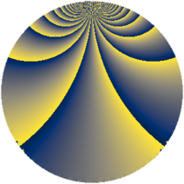# Properties

 Label 252.2.baLevel $252$ Weight $2$ Character orbit 252.ba Rep. character $\chi_{252}(155,\cdot)$ Character field $\Q(\zeta_{6})$ Dimension $72$ Newform subspaces $1$ Sturm bound $96$ Trace bound $0$

# Related objects

## Defining parameters

 Level: $$N$$ $$=$$ $$252 = 2^{2} \cdot 3^{2} \cdot 7$$ Weight: $$k$$ $$=$$ $$2$$ Character orbit: $$[\chi]$$ $$=$$ 252.ba (of order $$6$$ and degree $$2$$) Character conductor: $$\operatorname{cond}(\chi)$$ $$=$$ $$36$$ Character field: $$\Q(\zeta_{6})$$ Newform subspaces: $$1$$ Sturm bound: $$96$$ Trace bound: $$0$$

## Dimensions

The following table gives the dimensions of various subspaces of $$M_{2}(252, [\chi])$$.

Total New Old
Modular forms 104 72 32
Cusp forms 88 72 16
Eisenstein series 16 0 16

## Trace form

 $$72 q + 6 q^{6} + 4 q^{9} + O(q^{10})$$ $$72 q + 6 q^{6} + 4 q^{9} - 12 q^{12} - 34 q^{18} - 42 q^{20} - 2 q^{24} + 36 q^{25} + 28 q^{30} + 30 q^{32} - 44 q^{33} - 12 q^{34} + 20 q^{36} - 12 q^{40} - 60 q^{41} + 20 q^{42} - 24 q^{45} - 24 q^{46} - 28 q^{48} + 36 q^{49} - 78 q^{50} - 18 q^{52} - 10 q^{54} - 4 q^{57} - 18 q^{58} - 76 q^{60} - 60 q^{64} + 24 q^{65} + 54 q^{66} + 78 q^{68} + 24 q^{69} + 74 q^{72} - 24 q^{73} + 12 q^{76} - 20 q^{78} - 4 q^{81} - 36 q^{82} + 14 q^{84} + 30 q^{86} + 24 q^{88} + 8 q^{90} - 114 q^{92} - 96 q^{93} + 42 q^{94} + 112 q^{96} - 12 q^{97} + O(q^{100})$$

## Decomposition of $$S_{2}^{\mathrm{new}}(252, [\chi])$$ into newform subspaces

Label Dim $A$ Field CM Traces $q$-expansion
$a_{2}$ $a_{3}$ $a_{5}$ $a_{7}$
252.2.ba.a $72$ $2.012$ None $$0$$ $$0$$ $$0$$ $$0$$

## Decomposition of $$S_{2}^{\mathrm{old}}(252, [\chi])$$ into lower level spaces

$$S_{2}^{\mathrm{old}}(252, [\chi]) \cong$$ $$S_{2}^{\mathrm{new}}(36, [\chi])$$$$^{\oplus 2}$$